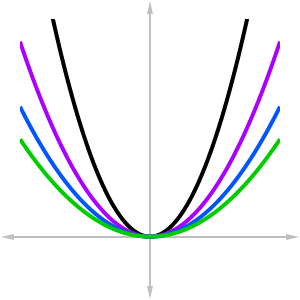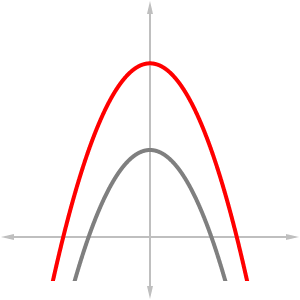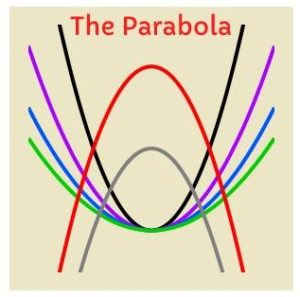## Putting It Together: Algebraic Operations on Functions

You have been tasked with creating a dazzling new logo for the school newspaper, The Parabola.  So of course you start thinking about how you might incorporate parabolas into the design.  The standard parabola, defined by the function  $f\left(x\right)=x^2$, is simply too boring by itself.  However by scaling, shifting, and reflecting, perhaps you might come up something more interesting.

First of all, the basic parabola shape seems too steep.  You can use a vertical compression to scale it down.  Remember, a vertical stretch or compression takes the form  $y=af\left(x\right)$, for a constant  $a$.

Vertical compression by a factor of 2:   $y={\Large\frac{1}{2}}x^2$

Vertical compression by a factor of 3:   $y={\Large\frac{1}{3}}x^2$

Vertical compression by a factor of 4:   $y={\Large\frac{1}{4}}x^2$

Let’s see what all of these functions look like when graphed together.  We’ll graph  $y=x^2$ in black, and then overlay the compressions by 2, 3, and 4, in purple, blue, and green, respectively.Not bad – in fact it’s looking like a pretty decent logo already, but let’s add a few more features.  The school colors are red and gray, so let’s put two upside-down parabolas in the foreground.  The transformation that reflects a graph about the $x$-axis is:  $y=f-\left(x^{ }\right)$.  But we also want to shift the graphs upwards after reflecting.  Therefore we should combine the reflection with a vertical shift.  Altogether, the two transformations are accomplished by  $y=-f\left(x\right)+k$ for a constant $k$.

Reflected and shifted up by 2:   $y=-x^2+2$

Reflected and shifted up by 4:   $y=-x^2+4$The red curve is $y=-x^2+4$, and the gray curve is $y=-x^2+2$.

Finally, let’s combine the parabolas all together and create our logo!  We’ll get rid of the axes, colorize the background, and include the name of the paper for our finished product.  Who knew that mathematics could help to create art?